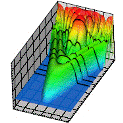## Research Papers in Physics and Astronomy## Anthony F. Starace Publications

2003

#### Comments

Published in Journal of Physics B: Atomic, Molecular and Optical Physics 36 (2003), pp. R49–R124. Copyright © 2003 Institute of Physics Publishing. Used by permission. http://www.iop.org/EJ/journal/JPhysB or http://stacks.iop.org/JPhysB/36/R49

#### Abstract

The quasistationary quasienergy state approach (QQES) is applied to the analysis of partial (n-photon) decay rates and angular distributions (ADs) of photoelectrons produced by an elliptically polarized laser field. The problem is formulated for a weakly bound electron with an energy E0 in the three-dimensional δ-model potential (which approximates the short-range potential of a negative ion) interacting with a strong monochromatic laser field having an electric vector F(ωt).The results presented cover weak (perturbative), strong (nonperturbative), and superstrong field regimes as well as a wide interval of frequencies ω extending from the tunnelling (ħω << |E0|) and multiphoton (ħω < |E0|) cases up to the high frequency domain (ħω > |E0|).

For a weak laser field, exact equations for the normalization factor and for the Fourier coefficients of the QQES wavefunction at the origin (|r| → 0) (that are key elements of the QQES approach for a δ-model potential) as well as for the detachment amplitudes are analyzed analytically using both standard Rayleigh–Schrödinger perturbation theory (PT) in the intensity, I, of the laser field and Brillouin–Wigner PT expansions involving the exact (complex) quasienergy ε. The lowest-order perturbative results for the n-photon ADs are presented in analytic form, and the parametrization of ADs in terms of polarization- and angular-independent atomic parameters is discussed for the general case of elliptical polarization. The major emphasis is on the analysis of an ellipticity induced distortion of three-dimensional ADs and, especially, on the elliptic dichroism (ED) effect, i.e., the dependence of the photoelectron yield in a fixed direction n on the sign of the ellipticity (or on the helicity) of a laser field. The dominant role of binding potential effects for a correct description of ED and threshold effects is demonstrated, and the intimate relationship between atomic ED factors and scattering phases of the detached electron is established for multiphoton detachment, including the above-threshold case.

For a strong laser field, we present an accurate derivation for the QQES wavefunction and decay rates in the Keldysh approximation (KA) from exact QQES equations, including analytical, first-order (“rescattering”) corrections to the KA results. The symmetries of ADs and the existence of ED are established using the exact analytical result for the n-photon detachment amplitude. Accurate numerical results are presented for the variation of the structure of the ADs as well as of the ED effect with increasing laser intensity.

For the high frequency case, ħω > |E0|, a rigorous analytical treatment of higher-order PT effects is presented for one-photon detachment, taking into account corrections of higher orders in I to thewell-known photodetachment cross section for a short-range potential. Together with the exact numerical analysis of the total and partial decay rates for ħω > > |E0|, these results demonstrate the existence of a quasistationary stabilization regime in the decay of a weakly bound electron for any polarization of the laser field. Moreover, this stabilization occurs only over a limited interval of intensity, up to the closure of the direct photodetachment channel.

In the superstrong field regime, the total decay rate of a weakly bound electron may be described by cycle-averaging the results for an instantaneous static electric field of strength |F(ωt)| (for any laser frequency and polarization). All results in this paper are presented in scaled units and are illustrated numerically for the case of the H negative ion.

COinS